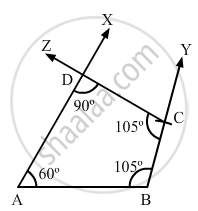Share

# Construct a Quadrilateral Abcd, Where Ab = 5.5 Cm, Bc = 3.7 Cm, ∠A = 60°, ∠B = 105° and ∠D = 90°. - Mathematics

Course
ConceptConstructing a Quadrilateral When Two Adjacent Sides and Three Angles Are Known

#### Question

Construct a quadrilateral ABCD, where AB = 5.5 cm, BC = 3.7 cm, ∠A = 60°, ∠B = 105° and ∠D = 90°.

#### Solution

We know that the sum of all the angles in a quadrilateral is 360 .

i . e . , ∠A + ∠B + ∠C + ∠D = 360°

⇒  ∠C = 105°

Steps of construction:

Step I: Draw AB = 5 . 5 cm .

Step II: Construct ∠XAB = 60° at A and ∠ABY = 105° .

Step III: With B as the centre and radius 3 . 7 cm, cut off BC = 3 . 7 cm .

Step IV: At C, draw ∠BCZ = 105° such that it meets AX at D .